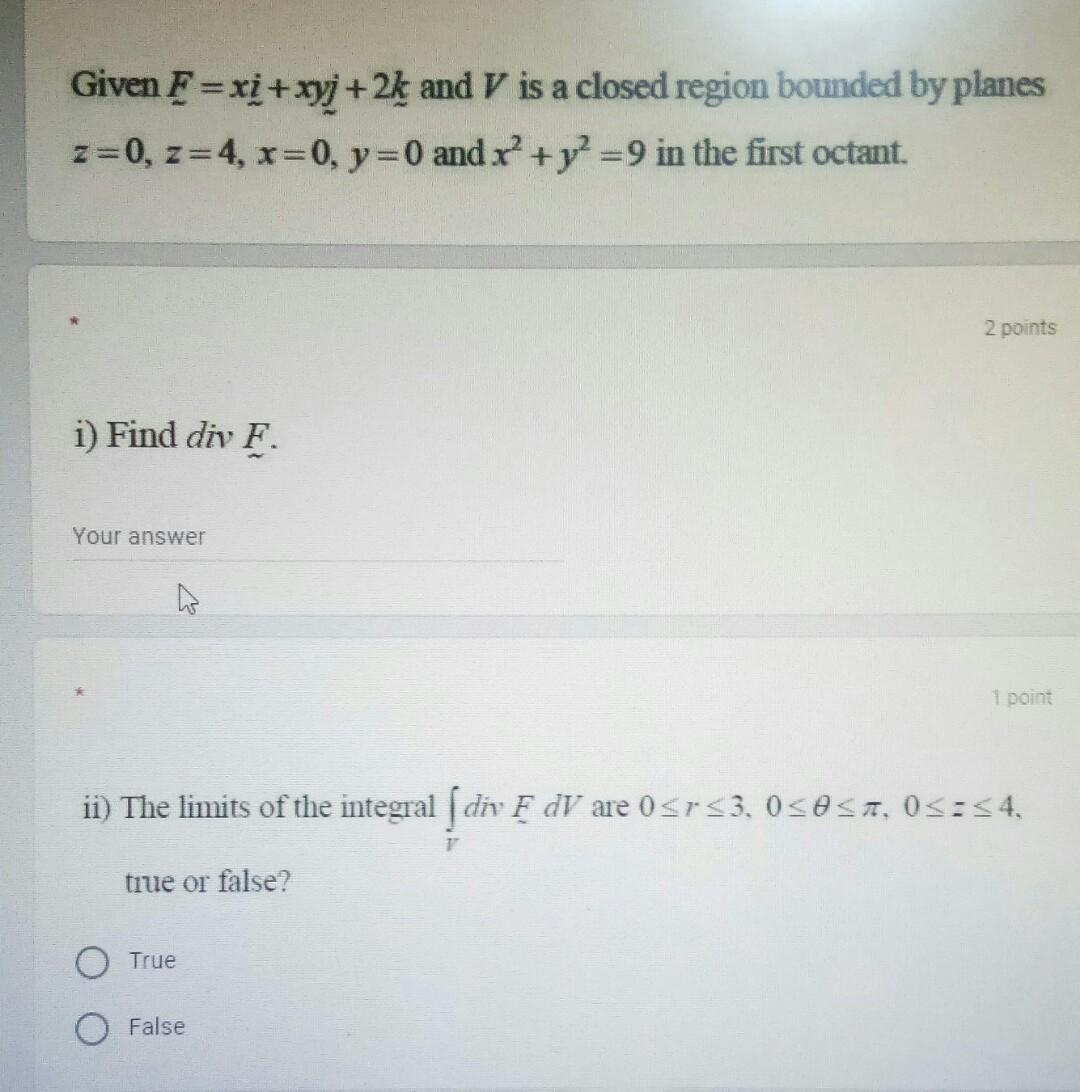### Create an Account

Already have account?

### Forgot Your Password ?

Home / Questions / Given F=xi + xyj +2k and V is a closed region bounded by planes z=0, z=4, x=0, y = 0 and x...

# Given F=xi + xyj +2k and V is a closed region bounded by planes z=0, z=4, x=0, y = 0 and x + y2 =9 in the first octant. 2 points i) Find div F. Your answer ho 1 point ii) The limits of the integral ſ

Given F=xi + xyj +2k and V is a closed region bounded by planes z=0, z=4, x=0, y = 0 and x + y2 =9 in the first octant. 2 points i) Find div F. Your answer ho 1 point ii) The limits of the integral ſ div F dV are o srs3, OSOSA, 03:54. 1 true or false? O True O FalseApr 14 2021 View more View Less

#### Answer (Solved)Subscribe To Get Solution